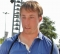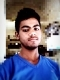## C++ Program to Check Prime Number By Creating a FunctionPublished on 27-Sep-2018 14:18:44
A prime number is a whole number that is greater than one and the only factors of a prime number should be one and itself.Some of the first prime numbers are −2, 3, 5, 7, 11, 13 ,17A program to check if a number is prime or not using a ... Read More

## C++ Program to Check Whether a Number is Palindrome or NotPublished on 27-Sep-2018 14:15:14
A palindrome number remains the same if its digits are reversed i.e its value does not change. A palindrome number can also be called symmetric. For example: The numbers 12321, 1551, 11 etc are palindromes as they do not change even if their digits are reversed.A program that checks if ... Read More

## C++ Program to Multiply two NumbersPublished on 27-Sep-2018 14:10:35
Multiplication of two numbers a and b yields their product. Value of a is added as many times as the value of b to get the product of a and b.For example.5 * 4 = 20 7 * 8 = 56 9 * 9 = 81Program to Multiply two Numbers ... Read More

## C++ Program to Find ASCII Value of a CharacterPublished on 27-Sep-2018 14:06:37
There are 128 characters in the ASCII (American Standard Code for Information Interchange) table with values ranging from 0 to 127.Some of the ASCII values of different characters are as follows −CharacterASCII ValueA65a97Z90z122\$36&38?63A program that finds the ASCII value of a character is given as follows −Example Live Demo#include <iostream> using ... Read MorePublished on 27-Sep-2018 13:50:02
Operator overloading can be done with most of the built-in operators in C++. The overloaded operators are functions with the keyword operator followed by the operator symbol that is defined. The overloaded operators have a return type and a parameter list like any function.A program that subtracts complex numbers using ... Read More

## C++ Program to Calculate Power of a NumberPublished on 27-Sep-2018 13:44:24
The power of a number can be calculated as x^y where x is the number and y is its power.For example.Let’s say, x = 2 and y = 10    x^y =1024    Here, x^y is 2^10 Power of a number can be calculated using recursive and non-recursive programs. Each ... Read More

## C++ Program to Reverse a NumberPublished on 27-Sep-2018 13:34:20
Reversing a number means storing its digits in reverse order.For example: If the number is 6529, then 9256 is displayed in the output.A program to reverse a number is given as follows −Example Live Demo#include <iostream> using namespace std; int main() {    int num = 63972, rev = 0;   ... Read More

## C++ Program to Find LCMPublished on 27-Sep-2018 13:27:52
The Least Common Multiple (LCM) of two numbers is the smallest number that is a multiple of both.For example: Let’s say we have the following two numbers: 15 and 9.15 = 5 * 3 9 = 3 * 3So, the LCM of 15 and 9 is 45.A program to find ... Read More

## C++ Program to Find GCDPublished on 27-Sep-2018 12:32:17
The Greatest Common Divisor (GCD) of two numbers is the largest number that divides both of them.For example: Let’s say we have two numbers are 45 and 27.45 = 5 * 3 * 3 27 = 3 * 3 * 3So, the GCD of 45 and 27 is 9.A program ... Read More

## C++ Program to Generate Multiplication TablePublished on 27-Sep-2018 12:25:35
The multiplication table is used to define a multiplication operation for any number. It is normally used to lay the foundation of elementary arithmetic operations with base ten numbers.The multiplication table of any number is written till 10. In each row, the product of the number with 1 to 10 ... Read More